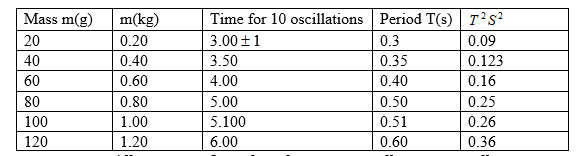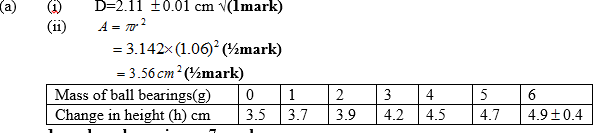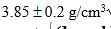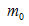##### Search### KCSE CLUSTER TESTS 23

#### Physics Paper 3

1.(f) - Correct labelling plus units. √ (1mark)

-Simple and uniform scale.√ (1mark)

-Plotting ½ mark each maximum.√ (2marks)

-Best line of fit through at least 3 correctly plotted points. √ (1mark)

(g) (i) P=slope.√ (1mark) correctly intervals from candidates graph.√ (1mark)

Accuracy 3.33 s2/kg1√ (1mark) (ii) Q=T2 –intercept.√ (1mark)

-correct value of Q read from candidates graph.√ (1mark) -accuracy 0.9+ 0.1√ (1mark)

20 marks

2.(d) (i) -Labelling of axis plus correct units.√ (1mark)

-Scale simple and uniform.√ (1mark)

-Plotting ½ each maximum.√ (2marks)

-Straight line passing through at least 3 correctly plotted points.√ (1mark)

-Correct intervals.√ (½mark)

-Correct evaluation to 2 d.p min. (½mark)

-Accuracy3√ (1 mark)

(ii)Intercept.√ (1 mark)

- Correctly read from the candidate’s graph.√ (1mark)

20 marks

Back Top